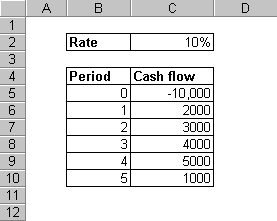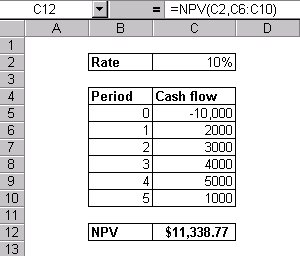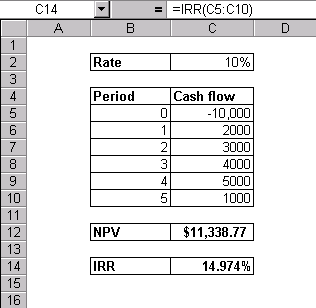# Calculating Net Present Value (NPV) and Internal Rate of Return (IRR) in Excel

### Net Present Value (NPV) Function

The NPV function calculates the present value of a series of cash flows at equal time intervals. The function is represented as follows:

= NPV(rate,value1,value2,...)
Here, rate is the discount rate for one period, and values are the cash flows. Any payments are entered with a negative sign, and income is entered as positive.

Note that even though the function is named Net Present Value (NPV), it doesn’t really calculate the net present value. This is because it does not take into consideration the initial investment at time 0.

To calculate the net present value, you will need to subtract the initial investment from the result you get from the NPV function.

Lets take an example to demonstrate this function. Assume that you started a business with an initial investment of $10,000 and received the following income for the next five years.To calculate the net present value, we will apply the NPV function as follows:This is the present value of all the future cash flows. The net present value will be: Net Present Value = 11,338.77 – 10,000 =$1,338.77

### Internal Rate of Return (IRR) Function

IRR is based on NPV. It as a special case of NPV, where the rate of return calculated is the interest rate corresponding to a 0 (zero) net present value.

IRR function is represented as follows:

= IRR(values,guess)

This function accounts for the inflows and the outflows, including the initial investment at time 0.

Using the same example above, the IRR calculation is shown below:The IRR of 14.974% means that at this rate the net present value will be zero.

### Other Related Functions

MIRR: MIRR calculates the modified internal rate of return for a series of periodic cash flows, considering both cost of investment and interest on reinvestment of cash.

Syntax: MIRR(values,finance_rate,investment_rate)

XNPV: This function calculates the net present value for a series of cash flows that are not equally spaced in time.

Syntax: XNPV(rate,values,dates)

XIRR: This function calculates the internal rate of return for a series of cash flows that are not equally spaced in time.

Syntax: XIRR(values,dates,guess)